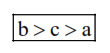Deepak Scored 45->99%ile with Bounce Back Crack Course. You can do it too!

# Arrange the following metal complex/ compounds in the increasing order of spin only magnetic moment.

Question:

Arrange the following metal complex/ compounds in the increasing order of spin only magnetic moment. Presume all the three, high spin system.

(Atomic numbers $\mathrm{Ce}=58, \mathrm{Gd}=64$ and $\mathrm{Eu}=63 .$ )

(a) $\left(\mathrm{NH}_{4}\right)_{2}\left[\mathrm{Ce}\left(\mathrm{NO}_{3}\right)_{6}\right]$

(b) $\mathrm{Gd}\left(\mathrm{NO}_{3}\right)_{3}$ and

(c) $\mathrm{Eu}\left(\mathrm{NO}_{3}\right)_{3}$

1. $(\mathrm{b})<(\mathrm{a})<(\mathrm{c})$

2. (c) $<(\mathrm{a})<(\mathrm{b})$

3. (a) $<(b)<(c)$

4. (a) $<($ c $)<($ b $)$

Correct Option: , 4

Solution:

(a) ${ }_{58} \mathrm{Ce} \rightarrow[\mathrm{Xe}] 4 \mathrm{f}^{2} 5 \mathrm{~d}^{0} 6 \mathrm{~s}^{2}$

In complex $\mathrm{Ce}^{4+} \rightarrow[\mathrm{Xe}] 4 \mathrm{f}^{0} 5 \mathrm{~d}^{0} 6 \mathrm{~s}^{0}$

there is no unpaired electron so $\mu_{\mathrm{m}}=0$

(b) ${ }_{64} \mathrm{Gd}^{3+} \rightarrow[\mathrm{Xe}] 4 \mathrm{f}^{7} 5 \mathrm{~d}^{0} 6 \mathrm{~s}^{0}$

contain seven unpaired electrons so,

$\mu_{\mathrm{m}}=\sqrt{7(7+2)}=\sqrt{63}$ B.M.

(c) ${ }_{63} \mathrm{Eu}^{3+} \rightarrow\left[{ }_{54} \mathrm{Xe}\right] 4 \mathrm{f}^{6} 5 \mathrm{~d}^{0} 6 \mathrm{~s}^{0}$

contain six unpaired electron

so, $\mu_{\mathrm{m}}=\sqrt{6(6+2)}=\sqrt{48}$ B.M.

Hence, order of spin only magnetic movement